# Norms¶

We now consider the different norms discussed in lecture:

$$||\mathbf{v}||_1 = \sum_{k=1}^n |v_k|$$$$||\mathbf{v}||_2 = \sqrt{\sum_{k=1}^n v_k^2}$$$$||\mathbf{v}||_\infty = \max |{v_k}|$$

We can use the inbuilt norm function to calculate norms:

In :
norm([1,2,3])==norm([1,2,3],2)==sqrt(1^2+2^2+3^2)

Out:
true
In :
norm([1,-2,3],1)==1+2+3

Out:
true
In :
norm([1,-2,3],Inf)==3

Out:
true

We will investigate these norms by plotting are the level curves for different norms. First, we discuss how to do a surface plot. The following plots $y*x^2$:

In :
f(x,y)=y*x^2

# this is short hand for
function f(x,y)
y*x^2
end

x=y=linspace(-1.,1.,100)

z=Float64[
f(x[j],y[k])
for k=1:length(y), j=1:length(x)]

using PyPlot

surf(x,y,z)  # 3D plot;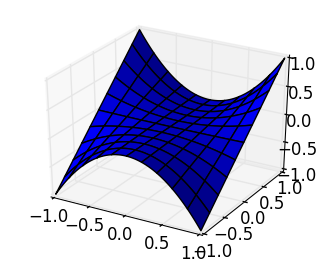Choosing f to be the 2-norm, we can see how the norm grows:

In :
f(x,y)=norm([x,y],2)

x=y=linspace(-1.,1.,200)

z=Float64[
f(x[j],y[k])
for k=1:length(y), j=1:length(x)]

using PyPlot

surf(x,y,z)  # 3D plot;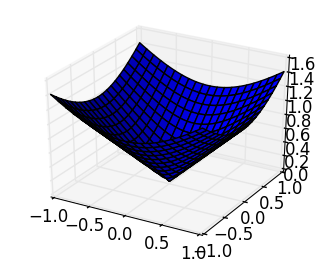It is helpful to plot a contour plot, to see where the curves of constant value are. Here, we see that the 2-norm forms circles:

In :
f(x,y)=norm([x,y],2)

x=y=linspace(-1.,1.,200)

z=Float64[
f(x[j],y[k])
for k=1:length(y), j=1:length(x)]

using PyPlot

contour(x,y,z)  # contour plot of f

gcf()[:set_size_inches](4,4)   # make the plot a square;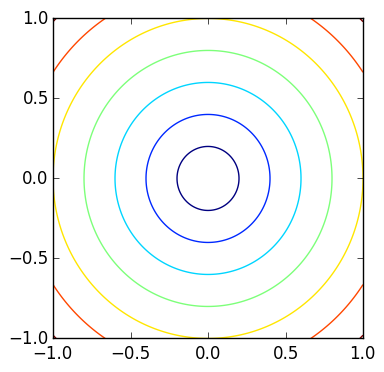The ∞-norm has squares of constant norm:

In :
f(x,y)=norm([x,y],Inf)

x=y=linspace(-1.,1.,200)

z=Float64[
f(x[j],y[k])
for k=1:length(y), j=1:length(x)]

using PyPlot

contour(x,y,z)  # 3D plot;

gcf()[:set_size_inches](4,4)   # make the plot a square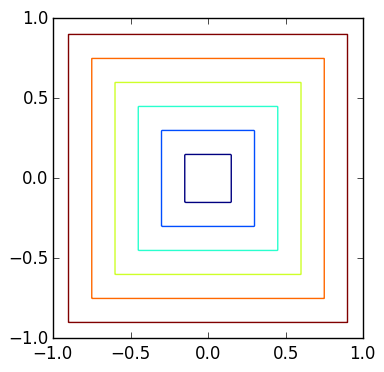The 1-norm has diamonds:

In :
f(x,y)=norm([x,y],1)

x=y=linspace(-1.,1.,200)

z=Float64[
f(x[j],y[k])
for k=1:length(y), j=1:length(x)]

using PyPlot

contour(x,y,z)  # 3D plot;

gcf()[:set_size_inches](4,4)   # make the plot a square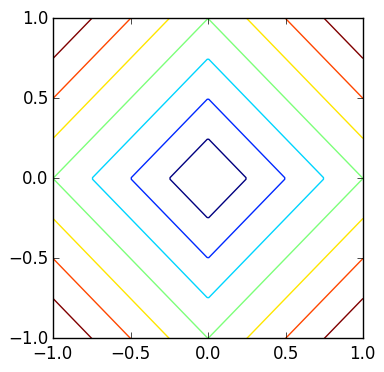More generally, the p-norm $$||\mathbf{v}||_p = \left(\sum_{k=1}^n |v_k|^p\right)^{1/p}$$ Is between a circle and a square:

In :
p=5

f(x,y)=norm([x,y],p)

x=y=linspace(-1.,1.,200)

z=Float64[
f(x[j],y[k])
for k=1:length(y), j=1:length(x)]

contour(x,y,z)  # 3D plot;
gcf()[:set_size_inches](4,4)   # make the plot a square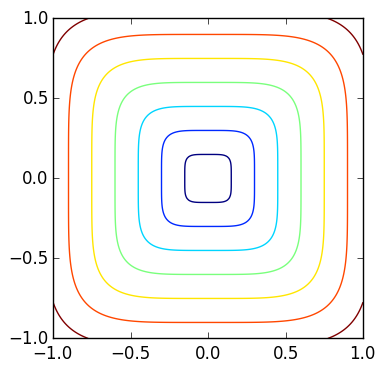We can also weight the norm using a diagonal matrix. For the 2-norm, this changes circles to ellipses:

In :
f(x,y)=norm([2 0; 0 1]*[x,y],2)

x=y=linspace(-1.,1.,200)

z=Float64[
f(x[j],y[k])
for k=1:length(y), j=1:length(x)]

contour(x,y,z)  # 3D plot;
gcf()[:set_size_inches](4,4)   # make the plot a square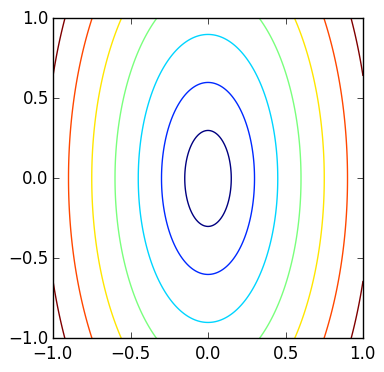# \ and least squares¶

x=A\b

will find x so that norm(A*x-b) is minimized: that is, we are finding the vector that finds the best approximation to the equation in the 2-norm. This is called the least squares solution.

In :
A=rand(10,5)
b=rand(10)

x=A\b

Out:
5-element Array{Float64,1}:
0.00826066
0.151079
0.881139
0.144133
0.350968  

We thus know norm(A*x-b) should be the smallest possible value achievable by any vector x.

In :
minnorm=norm(A*x-b)

Out:
0.4485301788323935

We can check that this is true by sampling many random vectors r, and double checking that norm(A*r-b) is greater than minnorm. This sort of experiment is known as Monte–Carlo simulation.

In :
randomminnorm=Inf   # start the smallest sampled norm at Inf

for k=1:1000000   # we will do a million trials
r=rand(5)    # create a random vector of size 5
newnrm=norm(A*r-b)     # Check ||A*r-b|| for  the random vector r r
randomminnorm = min(newnrm,randomminnorm)   # the minimal sample norm is the min
end
randomminnorm  # this is greater than minnorm

Out:
0.45086413589047813